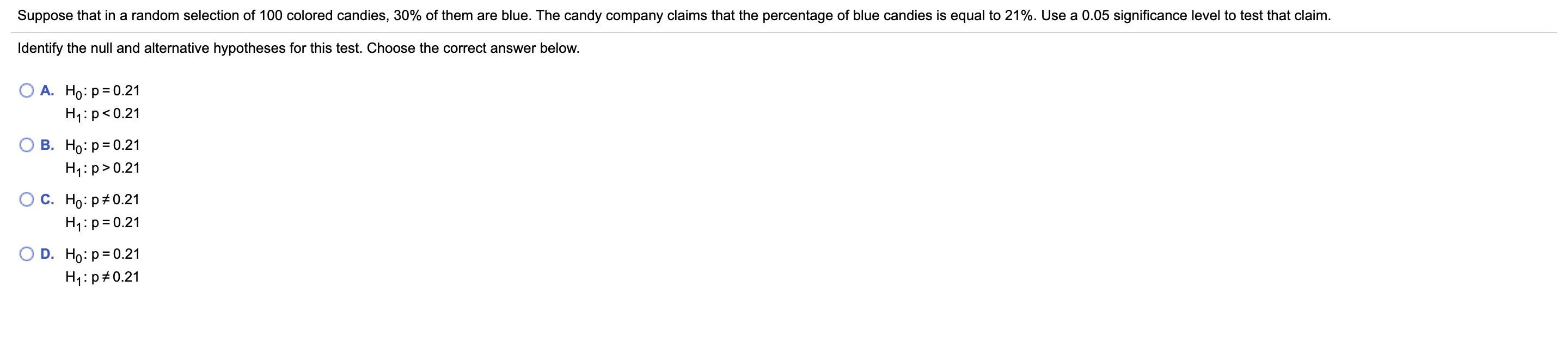# Suppose that in a random selection of 100 colored candies, 30% of them are blue. The candy company claims that the percentage of blue candies is equal to 21%. Use a 0.05 significance level to test that claim.Identify the null and alternative hypotheses for this test. Choose the correct answer below.А. Но: р30.21H1:p0.21С. Но: р#0.21H1:p= 0.21D. Ho: p= 0.21H1:p#0.21

Question
2 views

What is the test statistic and p-valuehelp_outlineImage TranscriptioncloseSuppose that in a random selection of 100 colored candies, 30% of them are blue. The candy company claims that the percentage of blue candies is equal to 21%. Use a 0.05 significance level to test that claim. Identify the null and alternative hypotheses for this test. Choose the correct answer below. А. Но: р30.21 H1:p<0.21 В. Но: Р3D0.21 H4:p>0.21 С. Но: р#0.21 H1:p= 0.21 D. Ho: p= 0.21 H1:p#0.21 fullscreen
check_circle

Step 1

Let p be the population proportion of blue candies.

A candy company claims that the percentage of blue candies is equal to 21%.

i.e., p =0.21

Step 2

Set up the hypotheses

Step 3

The test statistic is

where

The number of orders sampled n= 100

The sample proportion of blue c...

### Want to see the full answer?

See Solution

#### Want to see this answer and more?

Solutions are written by subject experts who are available 24/7. Questions are typically answered within 1 hour.*

See Solution
*Response times may vary by subject and question.
Tagged in

### Statistics